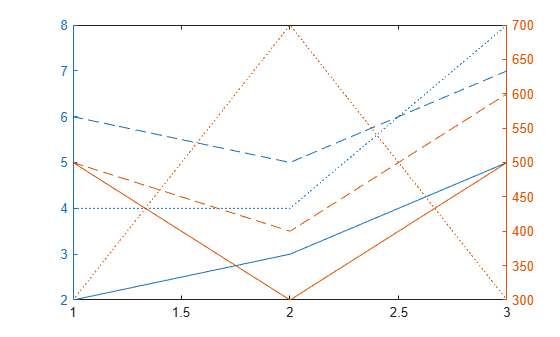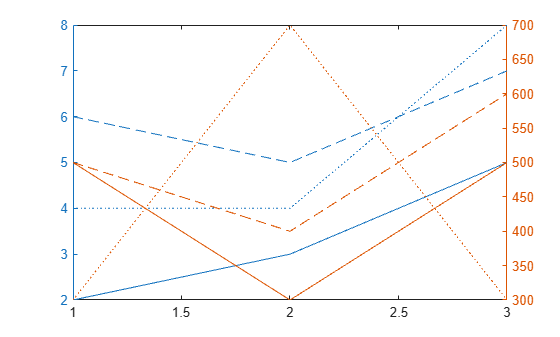## Modify Properties of Charts with Two y-Axes

The `yyaxis` function creates an `Axes` object with a y-axis on the left and right sides. Axes properties related to the y-axis have two values. However, MATLAB® gives access only to the value for the active side. For example, if the left side is active, then the `YDir` property of the `Axes` object contains the direction for the left y-axis. Similarly, if the right side is active, then the `YDir` property contains the direction for the right y-axis. An exception is that the `YAxis` property contains an array of two ruler objects (one for each y-axis).

You can change the appearance and behavior of a particular y-axis in either of these ways:

• Set the active side, and then change property values for the `Axes` object.

• Access the ruler objects through the `YAxis` property of the `Axes` object, and then change property values for the ruler object.

### Change Axes Properties

Modify properties of a chart with two y-axes by setting `Axes` properties.

Create a chart with two y-axes and plot data.

```x = [1 2 3]; y1 = [2 6 4; 3 5 4; 5 7 8]; y2 = 100*[5 5 3; 3 4 7; 5 6 3]; figure yyaxis left plot(x,y1) yyaxis right plot(x,y2)```Reverse the direction of increasing values along each y-axis. Use `yyaxis left` to activate the left side and set the direction for the left y-axis. Similarly, use `yyaxis right` to activate the right side. Then, set the direction for the right y-axis.

```ax = gca; yyaxis left ax.YDir = 'reverse'; yyaxis right ax.YDir = 'reverse';```### Change Ruler Properties

Modify properties of a chart with two y-axes by setting ruler properties.

Create a chart with two y-axes and plot data.

```x = [1 2 3]; y1 = [2 6 4; 3 5 4; 5 7 8]; y2 = 100*[5 5 3; 3 4 7; 5 6 3]; figure yyaxis left plot(x,y1) yyaxis right plot(x,y2)```Reverse the direction of increasing values along each y-axis by setting properties of the ruler object associated with each axis. Use `ax.YAxis(1)` to refer to the ruler for the left side and `ax.YAxis(2)` to refer to the ruler for the right side.

```ax = gca; ax.YAxis(1).Direction = 'reverse'; ax.YAxis(2).Direction = 'reverse';```### Specify Colors Using Default Color Order

Specify the colors for a chart with two y-axes by changing the default axes color order.

Create a figure. Define two RGB color values, one for the left side and one for the right side. Change the default axes color order to these two colors before creating the axes. Set the default value at the figure level so that the new colors affect only axes that are children of the figure `fig`. The new colors do not affect axes in other figures. Then create the chart.

```fig = figure; left_color = [.5 .5 0]; right_color = [0 .5 .5]; set(fig,'defaultAxesColorOrder',[left_color; right_color]); y = [1 2 3; 4 5 6]; yyaxis left plot(y) z = [6 5 4; 3 2 1]; yyaxis right plot(z)```